Variables Constants and Calculations Calculations Although the calculations

• Slides: 69Variables, Constants, and CalculationsCalculations • Although the calculations themselves are quite simple (addition, subtraction, multiplication, and division), there are some important issues to discuss first. • You must learn about variables and constants, the various types of data used by Visual C#, and how and where to declare variables and constants. • Variables are declared differently, depending on where you want to use them and how long you need to retain their values. • The code below is a small preview to show the calculation of the product of two text boxes. • The first group of statements declares the variables and their data types. • The second group of statements converts the text box contents to numeric and places the values into the variables. • The last line performs the multiplication and places the result into a variable.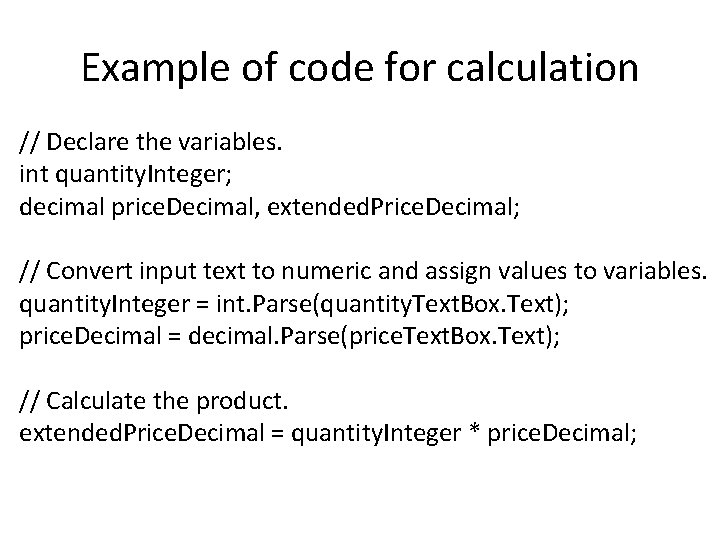Example of code for calculation // Declare the variables. int quantity. Integer; decimal price. Decimal, extended. Price. Decimal; // Convert input text to numeric and assign values to variables. quantity. Integer = int. Parse(quantity. Text. Box. Text); price. Decimal = decimal. Parse(price. Text. Box. Text); // Calculate the product. extended. Price. Decimal = quantity. Integer * price. Decimal;Data—Variables and Constants • So far, all data you have used in your projects have been properties of objects. • You have worked with the Text property of text boxes and labels. • Now you will work with values that are not properties. • C# allows you to set up locations in memory and give each location a name. • You can visualize each memory location as a scratch pad; the contents of the scratch pad can change as the need arises.Data—Variables and Constants • In this example, the memory location is called maximum. Integer = 100; • After executing this statement, the value of maximum. Integer is 100. • You can change the value of maximum. Integer, use it in calculations, or display it in a control. • In the preceding example, the memory location called maximum. Integer is a variable • Memory locations that hold data that can be changed during project execution are called variables ; locations that hold data that cannot change during execution are called constants. • For example, the customer’s name will vary as the information for each individual is processed. • However, the name of the company and the sales tax rate will remain the same (at least for that day). • When you declare a variable or a named constant , C# reserves an area of memory and assigns it a name, called an identifier. • You specify identifier names according to the rules of C# as well as some recommended naming conventions.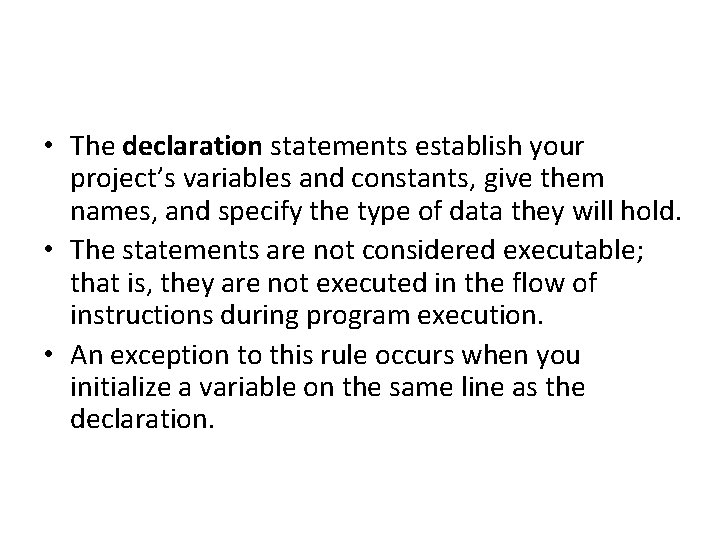• The declaration statements establish your project’s variables and constants, give them names, and specify the type of data they will hold. • The statements are not considered executable; that is, they are not executed in the flow of instructions during program execution. • An exception to this rule occurs when you initialize a variable on the same line as the declaration.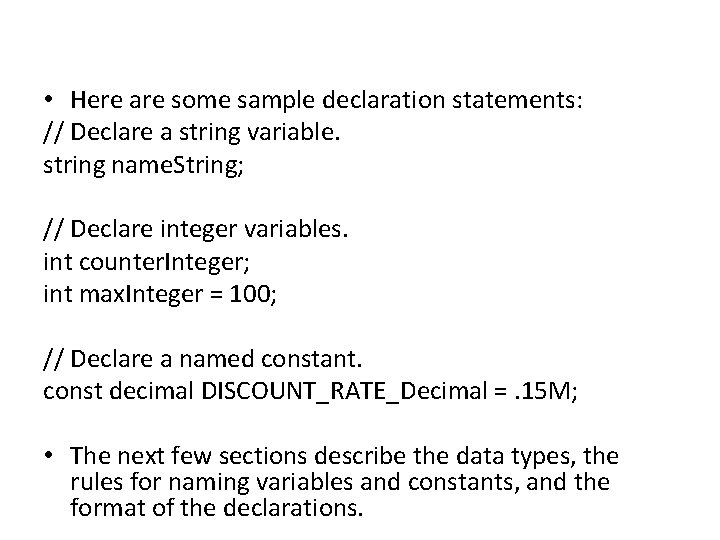• Here are some sample declaration statements: // Declare a string variable. string name. String; // Declare integer variables. int counter. Integer; int max. Integer = 100; // Declare a named constant. const decimal DISCOUNT_RATE_Decimal =. 15 M; • The next few sections describe the data types, the rules for naming variables and constants, and the format of the declarations.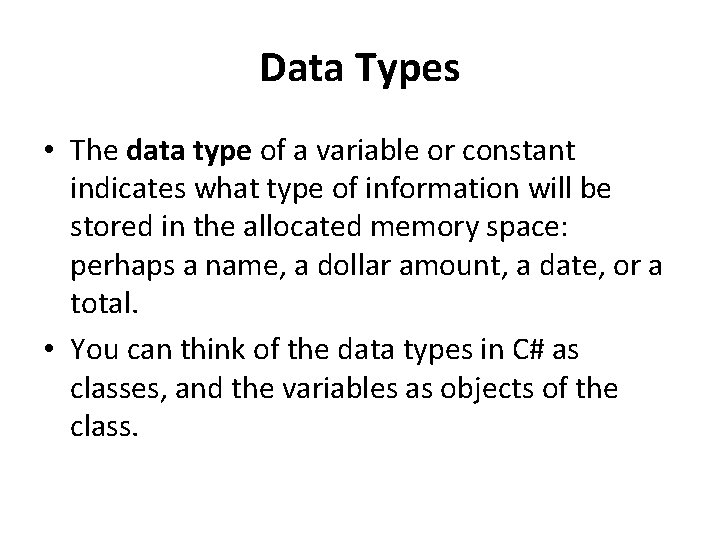Data Types • The data type of a variable or constant indicates what type of information will be stored in the allocated memory space: perhaps a name, a dollar amount, a date, or a total. • You can think of the data types in C# as classes, and the variables as objects of the class.The C# Data Types, . NET Common Language Runtime (CLR) Data Types, the Kind of Data Each Type Holds, and the Amount of Memory Allocated for Each.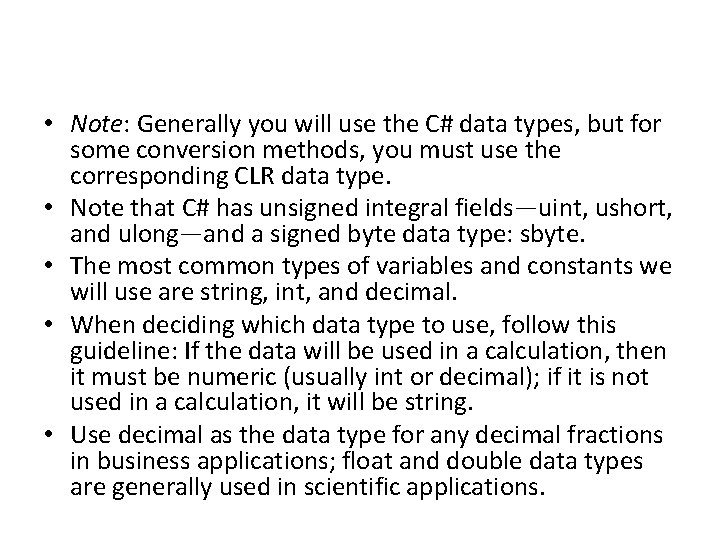• Note: Generally you will use the C# data types, but for some conversion methods, you must use the corresponding CLR data type. • Note that C# has unsigned integral fields—uint, ushort, and ulong—and a signed byte data type: sbyte. • The most common types of variables and constants we will use are string, int, and decimal. • When deciding which data type to use, follow this guideline: If the data will be used in a calculation, then it must be numeric (usually int or decimal); if it is not used in a calculation, it will be string. • Use decimal as the data type for any decimal fractions in business applications; float and double data types are generally used in scientific applications.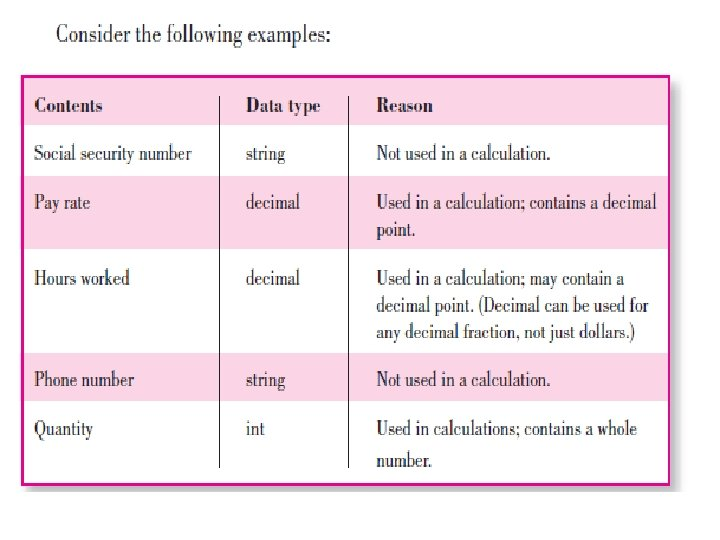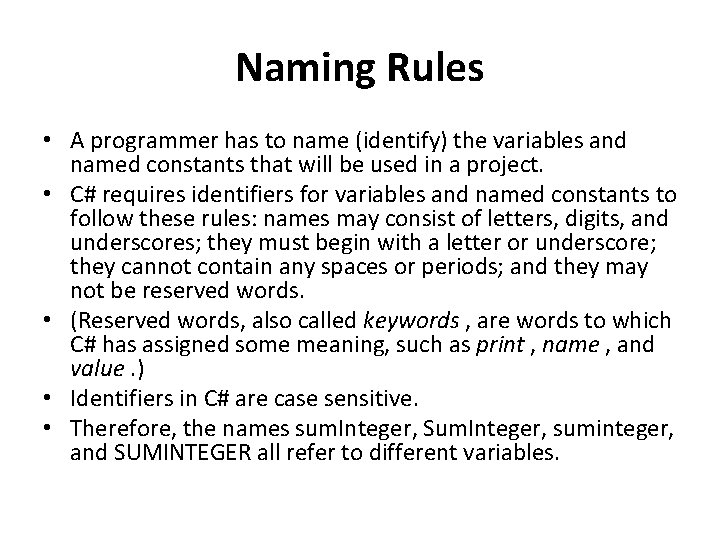Naming Rules • A programmer has to name (identify) the variables and named constants that will be used in a project. • C# requires identifiers for variables and named constants to follow these rules: names may consist of letters, digits, and underscores; they must begin with a letter or underscore; they cannot contain any spaces or periods; and they may not be reserved words. • (Reserved words, also called keywords , are words to which C# has assigned some meaning, such as print , name , and value. ) • Identifiers in C# are case sensitive. • Therefore, the names sum. Integer, Sum. Integer, suminteger, and SUMINTEGER all refer to different variables.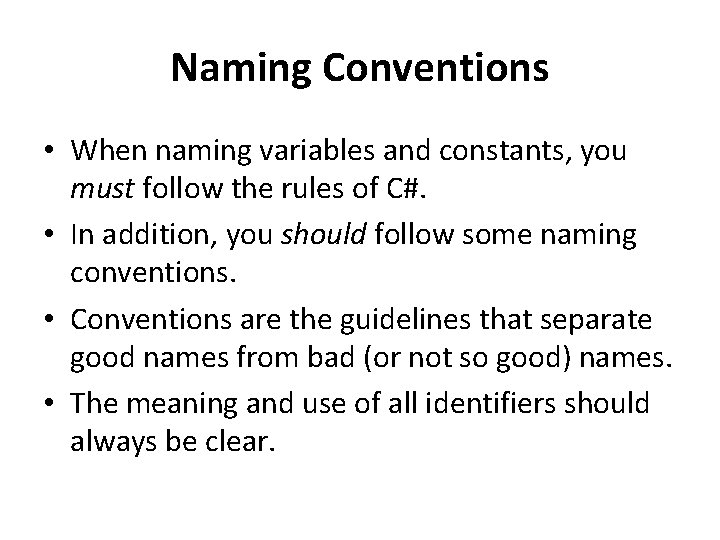Naming Conventions • When naming variables and constants, you must follow the rules of C#. • In addition, you should follow some naming conventions. • Conventions are the guidelines that separate good names from bad (or not so good) names. • The meaning and use of all identifiers should always be clear.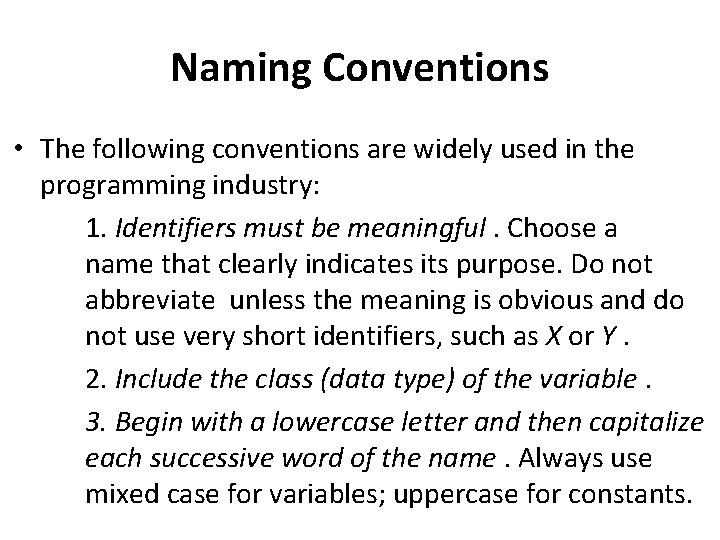Naming Conventions • The following conventions are widely used in the programming industry: 1. Identifiers must be meaningful. Choose a name that clearly indicates its purpose. Do not abbreviate unless the meaning is obvious and do not use very short identifiers, such as X or Y. 2. Include the class (data type) of the variable. 3. Begin with a lowercase letter and then capitalize each successive word of the name. Always use mixed case for variables; uppercase for constants.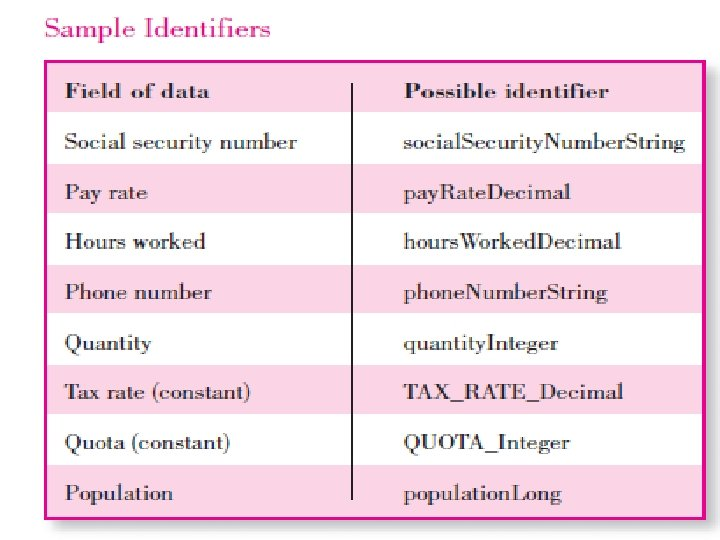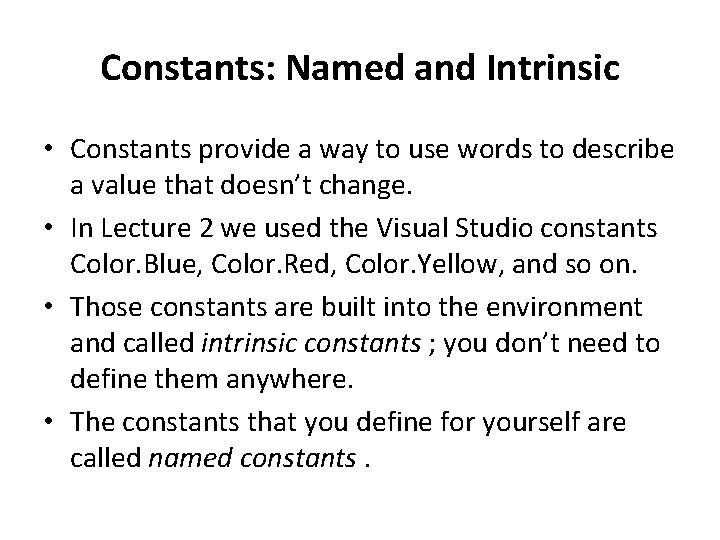Constants: Named and Intrinsic • Constants provide a way to use words to describe a value that doesn’t change. • In Lecture 2 we used the Visual Studio constants Color. Blue, Color. Red, Color. Yellow, and so on. • Those constants are built into the environment and called intrinsic constants ; you don’t need to define them anywhere. • The constants that you define for yourself are called named constants.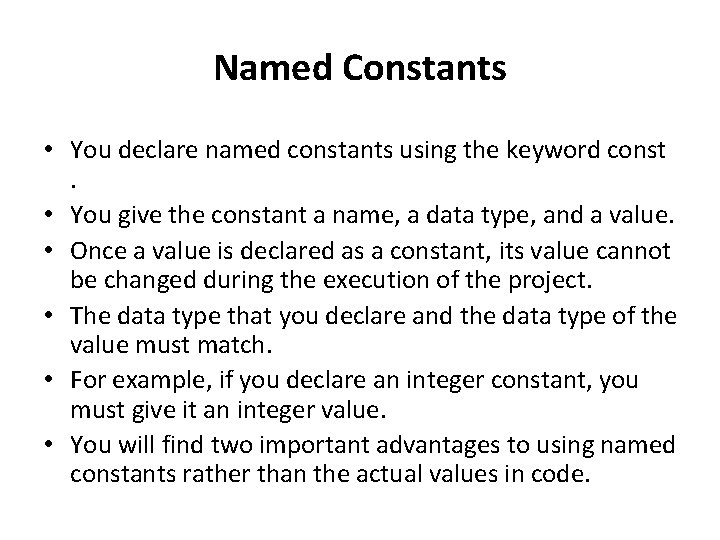Named Constants • You declare named constants using the keyword const. • You give the constant a name, a data type, and a value. • Once a value is declared as a constant, its value cannot be changed during the execution of the project. • The data type that you declare and the data type of the value must match. • For example, if you declare an integer constant, you must give it an integer value. • You will find two important advantages to using named constants rather than the actual values in code.Named Constants • The code is easier to read; for example, seeing the identifier MAXIMUM_PAY_Decimal is more meaningful than seeing a number such as 1000. • In addition, if you need to change the value at a later time, you need to change the constant declaration only once; you do not have to change every reference to it throughout the code.const Statement—General Form • const Datatype Identifier = Value ; • Naming conventions for constants require that you include the data type in the name. • Use all uppercase for the name with individual words separated by underscores.• This example sets the company name, address, and the sales tax rate as constants: • const Statement—Examples const string COMPANY_NAME_String = "R 'n R -- for Reading 'n Refreshment"; const string COMPANY_ADDRESS_String = "101 S. Main Street"; const decimal SALES_TAX_RATE_Decimal =. 08 m;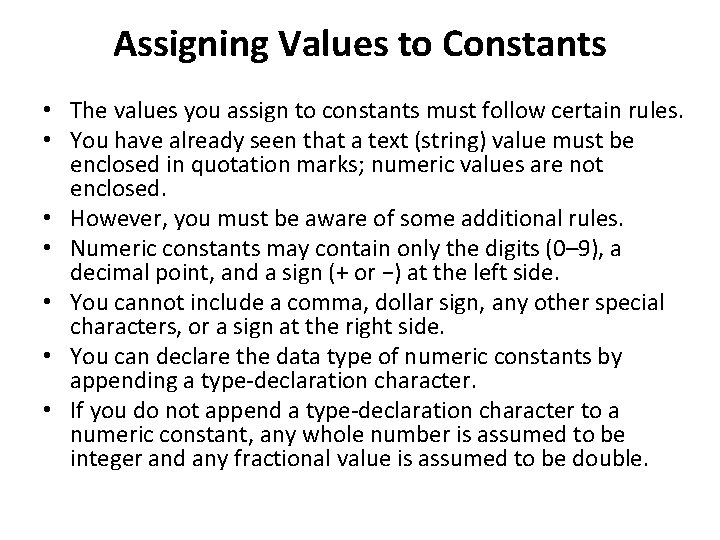Assigning Values to Constants • The values you assign to constants must follow certain rules. • You have already seen that a text (string) value must be enclosed in quotation marks; numeric values are not enclosed. • However, you must be aware of some additional rules. • Numeric constants may contain only the digits (0– 9), a decimal point, and a sign (+ or −) at the left side. • You cannot include a comma, dollar sign, any other special characters, or a sign at the right side. • You can declare the data type of numeric constants by appending a type-declaration character. • If you do not append a type-declaration character to a numeric constant, any whole number is assumed to be integer and any fractional value is assumed to be double.Assigning Values to Constants • The type-declaration characters are decimal M or m double D or d long L or l short S or s float F or f • String literals (also called string constants) may contain letters, digits, and special characters such as \$#@%&*. • You will have a problem when you want to include quotation marks inside a string literal since quotation marks enclose the literal. • The solution is to precede the quotation mark with a backslash (), which specifies that the character following should be rendered as is.• Example "He said, "I like it. " " produces this string: He said, "I like it. “ • You can use two backslashes when you need to include a backslash in a string literal. • The first backslash specifies that you want the following character rendered as it appears. • Example string file. Path. String = "C: \Personal. Documents\Personal. Letter";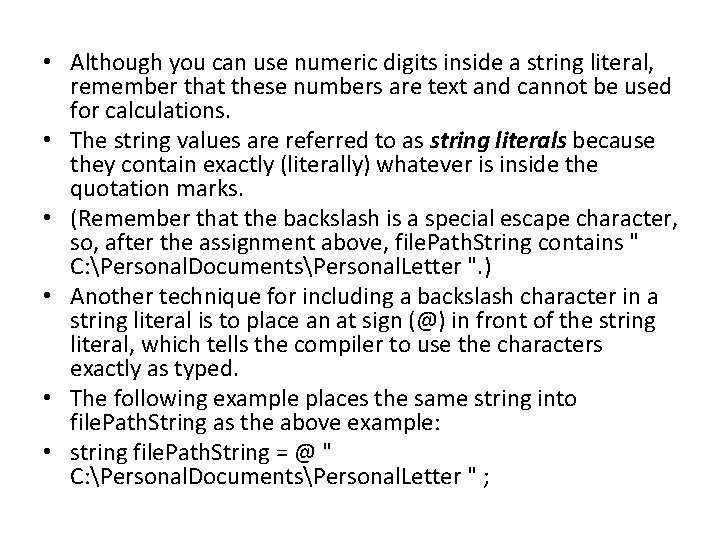• Although you can use numeric digits inside a string literal, remember that these numbers are text and cannot be used for calculations. • The string values are referred to as string literals because they contain exactly (literally) whatever is inside the quotation marks. • (Remember that the backslash is a special escape character, so, after the assignment above, file. Path. String contains " C: Personal. DocumentsPersonal. Letter ". ) • Another technique for including a backslash character in a string literal is to place an at sign (@) in front of the string literal, which tells the compiler to use the characters exactly as typed. • The following example places the same string into file. Path. String as the above example: • string file. Path. String = @ " C: Personal. DocumentsPersonal. Letter " ;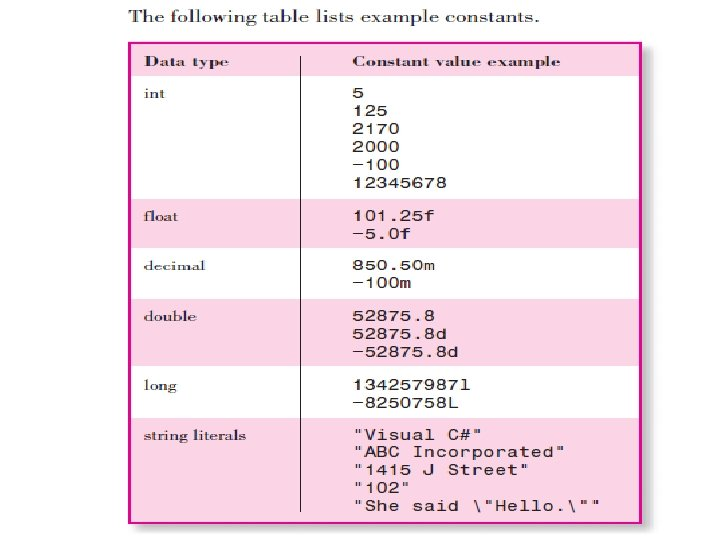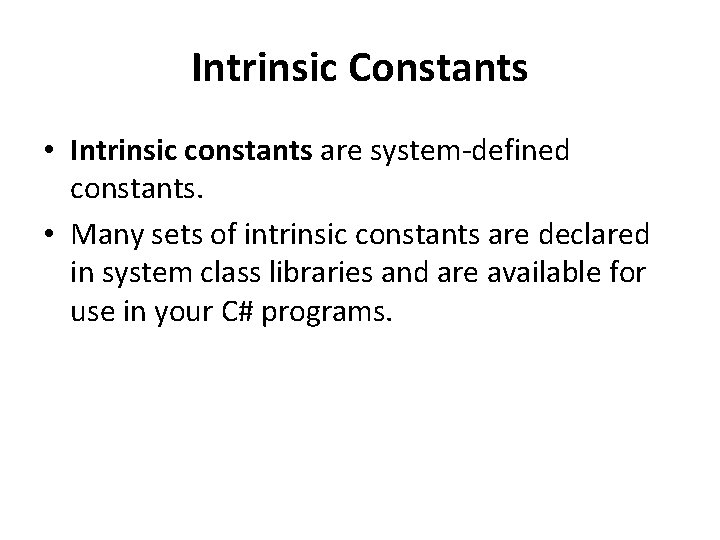Intrinsic Constants • Intrinsic constants are system-defined constants. • Many sets of intrinsic constants are declared in system class libraries and are available for use in your C# programs.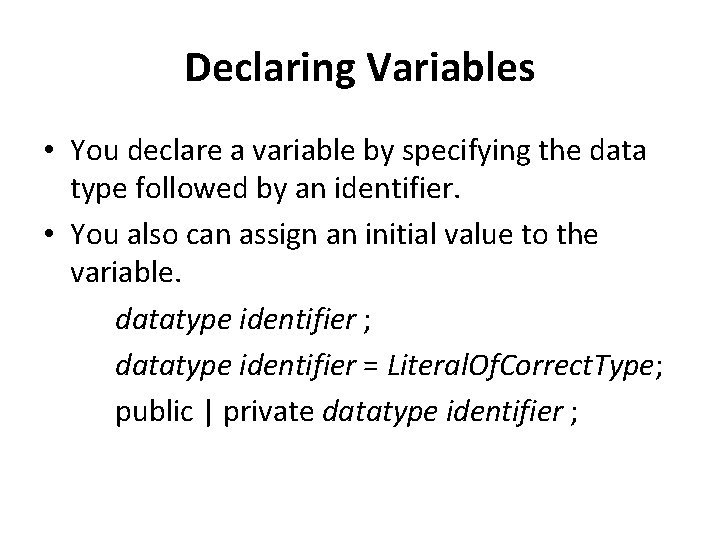Declaring Variables • You declare a variable by specifying the data type followed by an identifier. • You also can assign an initial value to the variable. datatype identifier ; datatype identifier = Literal. Of. Correct. Type; public | private datatype identifier ;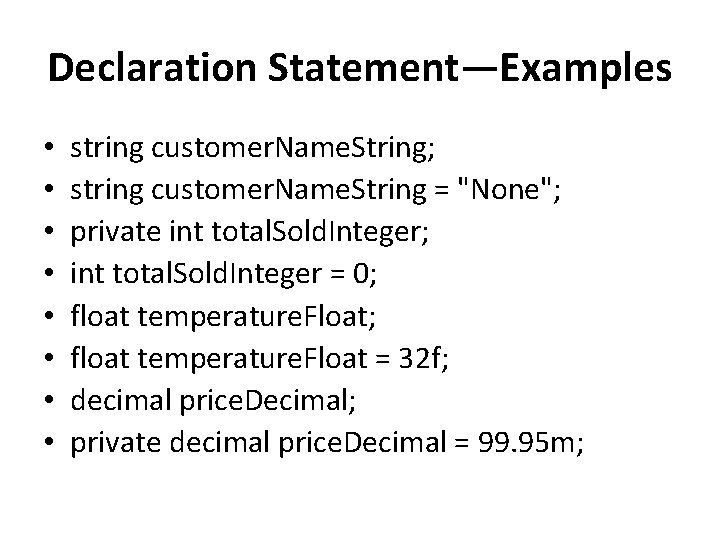Declaration Statement—Examples • • string customer. Name. String; string customer. Name. String = "None"; private int total. Sold. Integer; int total. Sold. Integer = 0; float temperature. Float = 32 f; decimal price. Decimal; private decimal price. Decimal = 99. 95 m;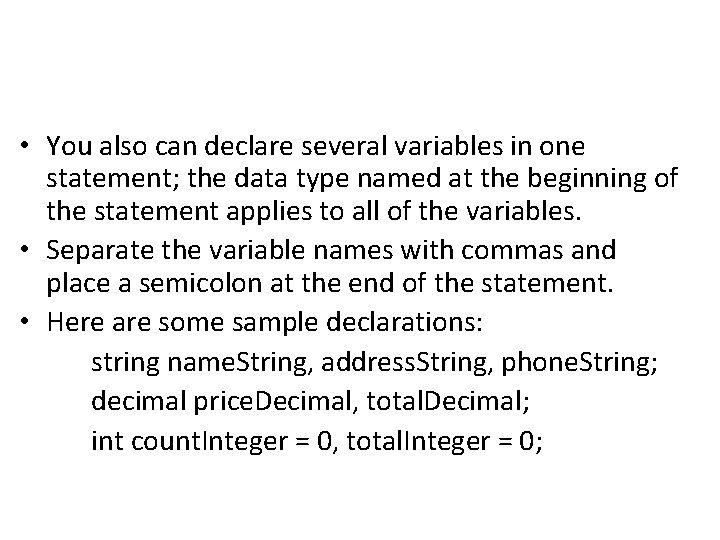• You also can declare several variables in one statement; the data type named at the beginning of the statement applies to all of the variables. • Separate the variable names with commas and place a semicolon at the end of the statement. • Here are some sample declarations: string name. String, address. String, phone. String; decimal price. Decimal, total. Decimal; int count. Integer = 0, total. Integer = 0;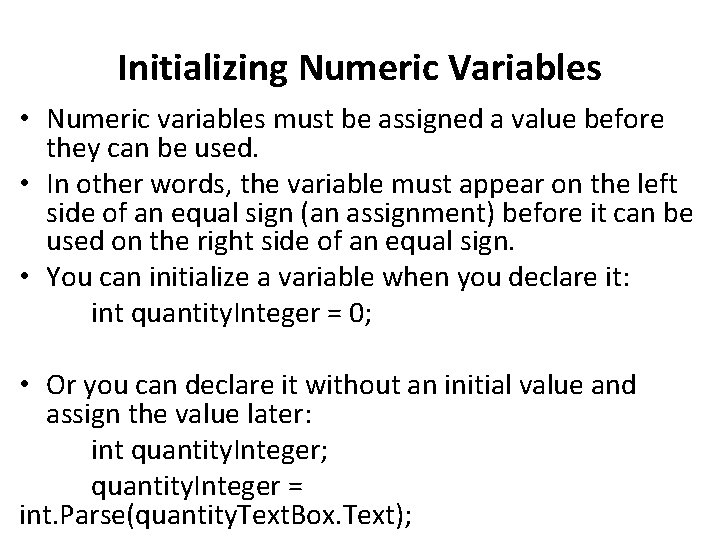Initializing Numeric Variables • Numeric variables must be assigned a value before they can be used. • In other words, the variable must appear on the left side of an equal sign (an assignment) before it can be used on the right side of an equal sign. • You can initialize a variable when you declare it: int quantity. Integer = 0; • Or you can declare it without an initial value and assign the value later: int quantity. Integer; quantity. Integer = int. Parse(quantity. Text. Box. Text);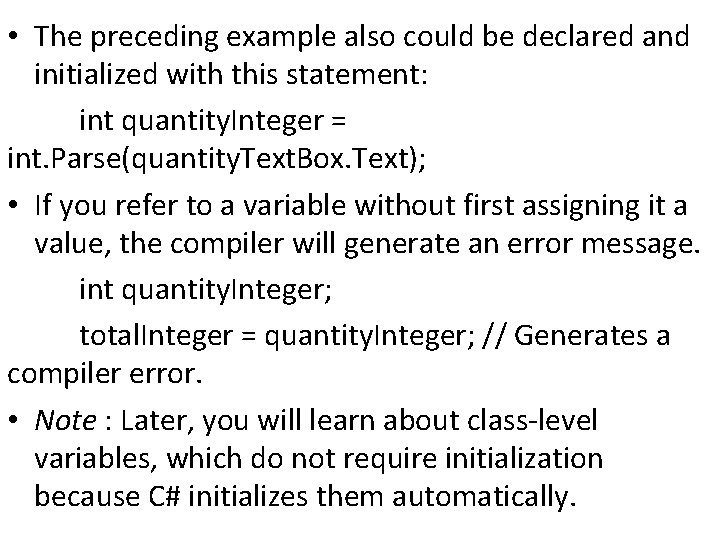• The preceding example also could be declared and initialized with this statement: int quantity. Integer = int. Parse(quantity. Text. Box. Text); • If you refer to a variable without first assigning it a value, the compiler will generate an error message. int quantity. Integer; total. Integer = quantity. Integer; // Generates a compiler error. • Note : Later, you will learn about class-level variables, which do not require initialization because C# initializes them automatically.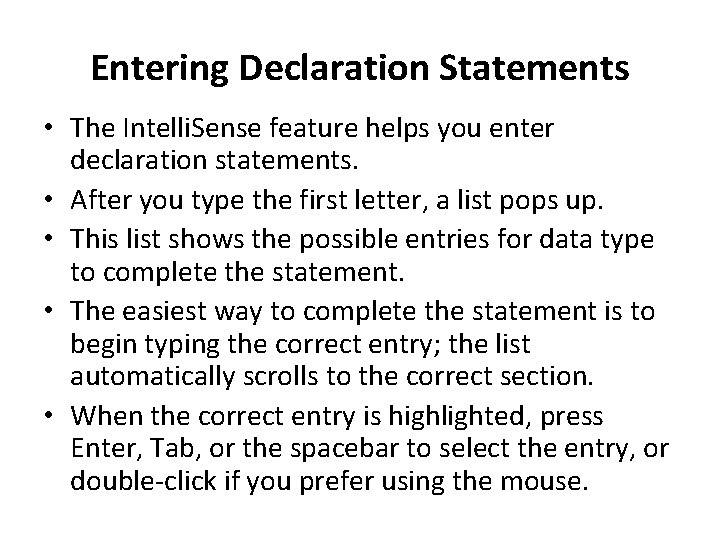Entering Declaration Statements • The Intelli. Sense feature helps you enter declaration statements. • After you type the first letter, a list pops up. • This list shows the possible entries for data type to complete the statement. • The easiest way to complete the statement is to begin typing the correct entry; the list automatically scrolls to the correct section. • When the correct entry is highlighted, press Enter, Tab, or the spacebar to select the entry, or double-click if you prefer using the mouse.• Note : Some people find the Intelli. Sense feature annoying rather than helpful. • You can turn off the feature by selecting Tools / Options. • In the Options dialog box, choose T ext Editor / C# / General ; deselect A uto list m embers and Parameter information. • If you are using the C# Express Edition, you must select Show all settings in the Options dialog box to make the selections.Scope and Lifetime of Variables • A variable may exist and be visible for all classes in a project, a single class, a single form (which is a class), a single method in a class, or inside a single block in a method. • The visibility of a variable is referred to as its scope. • Visibility really means “this variable can be used or ‘seen’ in this location. ” • The scope is said to be namespace, class level, local, or block. • A namespace variable may be used in all methods of the namespace, which is generally the entire project. • Class-level variables are accessible in all methods of a form or other class. • A local variable may be used only within the method in which it is declared, and a block variable is used only within a block of code inside a method. • A block is defined as the code enclosed in curly braces. • You declare the scope of a variable by choosing where to declare it. • Note : Some programming languages and some programmers refer to namespace variables as global variables.Variable Lifetime • When you create a variable, you must be aware of its lifetime. • The lifetime of a variable is the period of time that the variable exists. • The lifetime of a local or block variable is normally one execution of a method. • For example, each time you execute a method, the local declarations are executed. • Each variable is created as a “fresh” new one, with the initial value that you assign for it. • When the method finishes, its variables disappear; that is, their memory locations are released. • The lifetime of a class-level variable is the entire time the class is loaded, generally the lifetime of the entire project. • If you want to maintain the value of a variable for multiple executions of a method, for example, to calculate a running total, you must use a class-level variable (or a variable declared as static)Local Declarations • Any variable that you declare inside a method is local in scope, which means that it is known only to that method. • The keywords public and private are not used on local variables. • A declaration may appear anywhere inside the method as long as it appears prior to the first use of the variable in a statement. • However, good programming practices dictate that all declarations appear at the top of the method, prior to all other code statements (after the comments).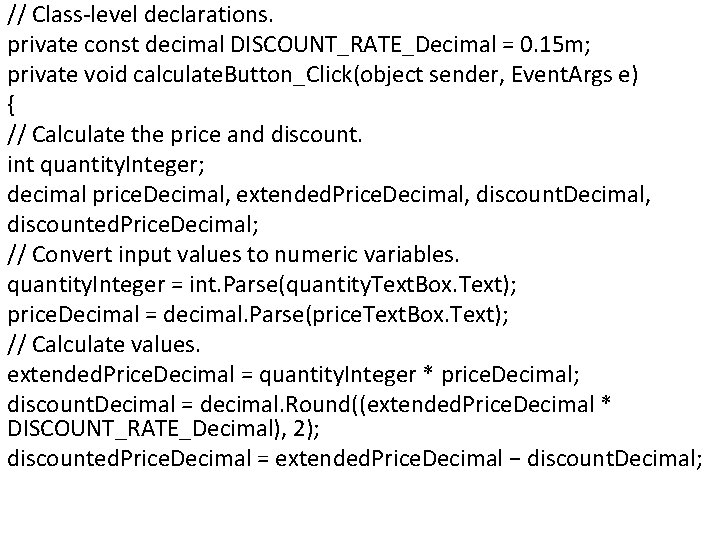// Class-level declarations. private const decimal DISCOUNT_RATE_Decimal = 0. 15 m; private void calculate. Button_Click(object sender, Event. Args e) { // Calculate the price and discount. int quantity. Integer; decimal price. Decimal, extended. Price. Decimal, discounted. Price. Decimal; // Convert input values to numeric variables. quantity. Integer = int. Parse(quantity. Text. Box. Text); price. Decimal = decimal. Parse(price. Text. Box. Text); // Calculate values. extended. Price. Decimal = quantity. Integer * price. Decimal; discount. Decimal = decimal. Round((extended. Price. Decimal * DISCOUNT_RATE_Decimal), 2); discounted. Price. Decimal = extended. Price. Decimal − discount. Decimal;• Notice the private const statement in the preceding example. • Although you can declare named constants to be local, block level, class level, or namespace in scope, just as you can variables, good programming practices dictate that constants should be declared at the class level. • This technique places all constant declarations at the top of the code and makes them easy to find in case you need to make changes.Class-Level Declarations • At times you need to be able to use a variable or constant in more than one method of a form. • When you declare a variable or constant as class level, you can use it anywhere in that form’s class. • When you write class-level declarations, you can use the public or private keywords or just use the data type. • Good programming practices dictate that you use the private keyword unless you need a variable to be public. • Place the declarations for class-level variables and constants after the opening brace for the class, outside of any method. I • f you wish to accumulate a sum or count items for multiple executions of a method, you should declare the variable at the class level.Coding Class-Level Declarations • To enter class-level declarations, place the insertion point on a new line after the class declaration and its opening curly brace. • Declare the variables and constants after the class declaration but before your first method.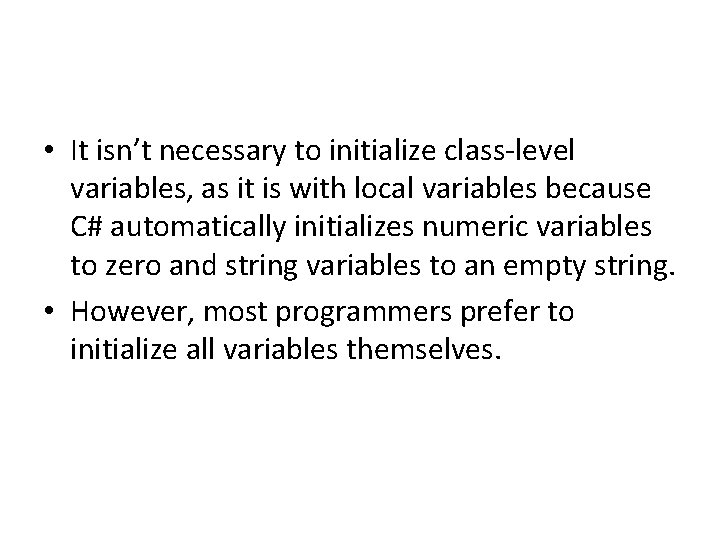• It isn’t necessary to initialize class-level variables, as it is with local variables because C# automatically initializes numeric variables to zero and string variables to an empty string. • However, most programmers prefer to initialize all variables themselves.Block-Level and Namespace-Level Declarations • Block-level variables and constants have a scope of a block of code, i. e. the code contained within a pair of curly braces ({}). • These statements are covered later • Namespace-level variables and constants can sometimes be useful when a project has multiple forms and/or classes, but good programming practices exclude the use of namespace-level variables.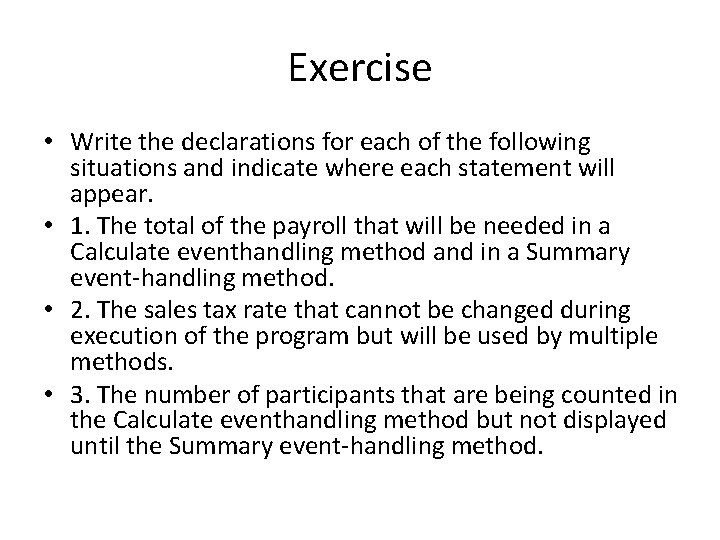Exercise • Write the declarations for each of the following situations and indicate where each statement will appear. • 1. The total of the payroll that will be needed in a Calculate eventhandling method and in a Summary event-handling method. • 2. The sales tax rate that cannot be changed during execution of the program but will be used by multiple methods. • 3. The number of participants that are being counted in the Calculate eventhandling method but not displayed until the Summary event-handling method.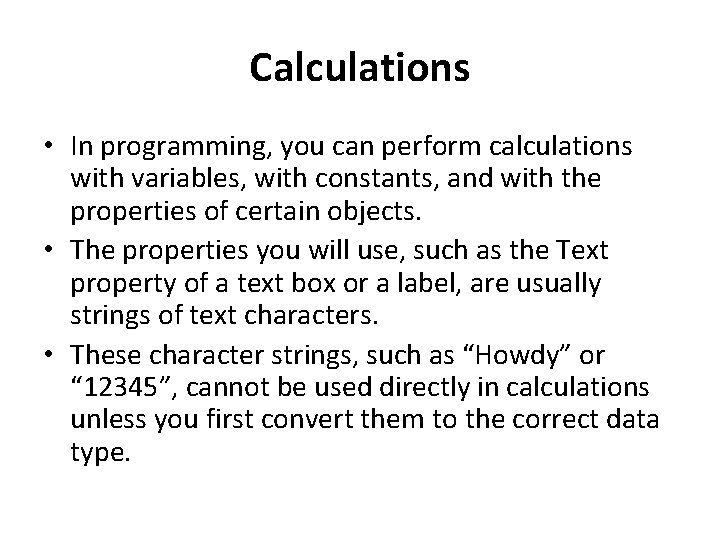Calculations • In programming, you can perform calculations with variables, with constants, and with the properties of certain objects. • The properties you will use, such as the Text property of a text box or a label, are usually strings of text characters. • These character strings, such as “Howdy” or “ 12345”, cannot be used directly in calculations unless you first convert them to the correct data type.Converting Strings to a Numeric Data Type • You can use a Parse method to convert the Text property of a control to its numeric form before you use the value in a calculation. • The class that you use depends on the data type of the variable to which you are assigning the value. • For example, to convert text to an integer, use the int. Parse method; to convert to a decimal value, use decimal. Parse. • Pass the text string that you want to convert as an argument of the Parse method.Example • // Convert input values to numeric variables. • quantity. Integer = int. Parse(quantity. Text. Box. Text); • price. Decimal = decimal. Parse(price. Text. Box. Text); • // Calculate the extended price. • extended. Price. Decimal = quantity. Integer * price. Decimal;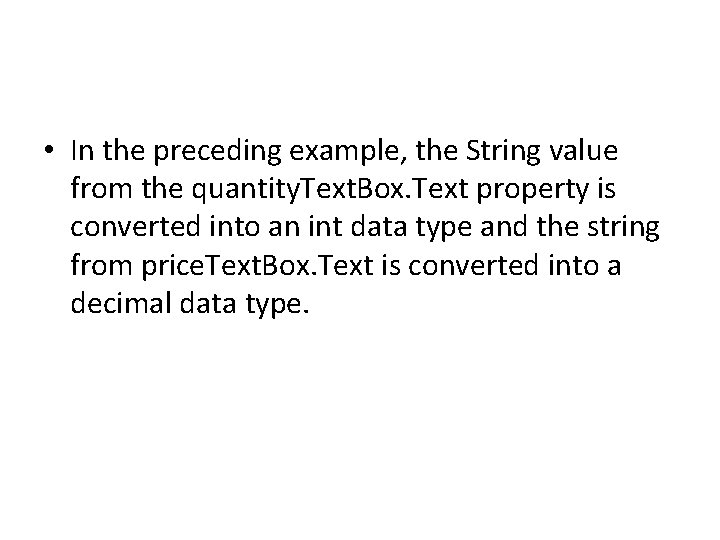• In the preceding example, the String value from the quantity. Text. Box. Text property is converted into an int data type and the string from price. Text. Box. Text is converted into a decimal data type.Using the Parse Methods • As you know, objects have methods that perform actions, such as the Focus method for a text box. • The data types that you use to declare variables are classes, which have properties and methods. • Each of the numeric data type classes has a Parse method, which you will use to convert text strings into the correct numeric value for that type. • The decimal class has a Parse method that converts the value inside the parentheses to a decimal value while the int class has a Parse method to convert the value to an integer.The Parse Methods—General Form • // Convert to int. • int. Parse( String. To. Convert ); • // Convert to decimal. • decimal. Parse( String. To. Convert ) ; • The expression you wish to convert can be the property of a control, a string variable, or a string constant. • The Parse method returns (produces) a value that can be used as a part of a statement, such as the assignment statements in the following examples.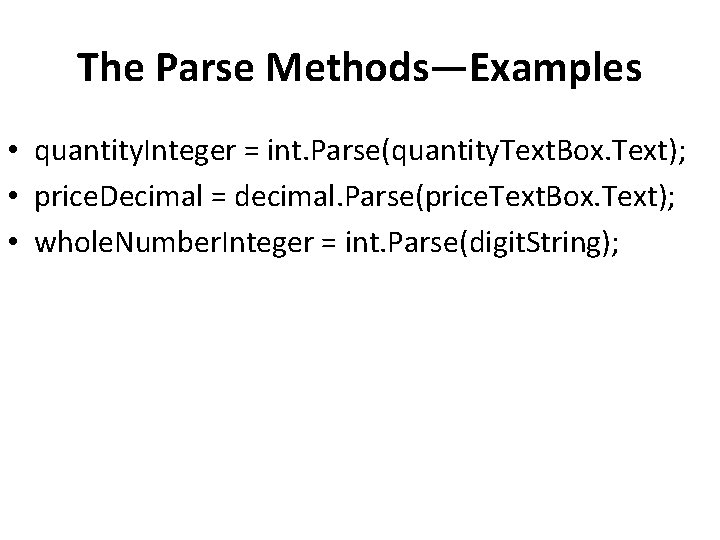The Parse Methods—Examples • quantity. Integer = int. Parse(quantity. Text. Box. Text); • price. Decimal = decimal. Parse(price. Text. Box. Text); • whole. Number. Integer = int. Parse(digit. String);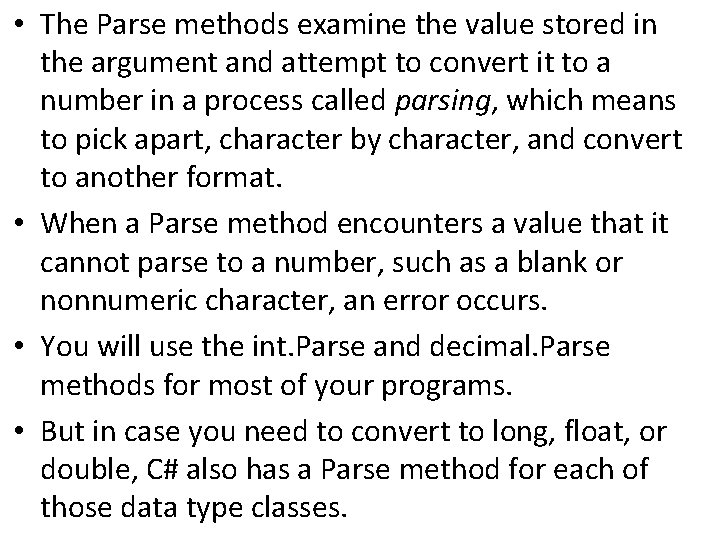• The Parse methods examine the value stored in the argument and attempt to convert it to a number in a process called parsing, which means to pick apart, character by character, and convert to another format. • When a Parse method encounters a value that it cannot parse to a number, such as a blank or nonnumeric character, an error occurs. • You will use the int. Parse and decimal. Parse methods for most of your programs. • But in case you need to convert to long, float, or double, C# also has a Parse method for each of those data type classes.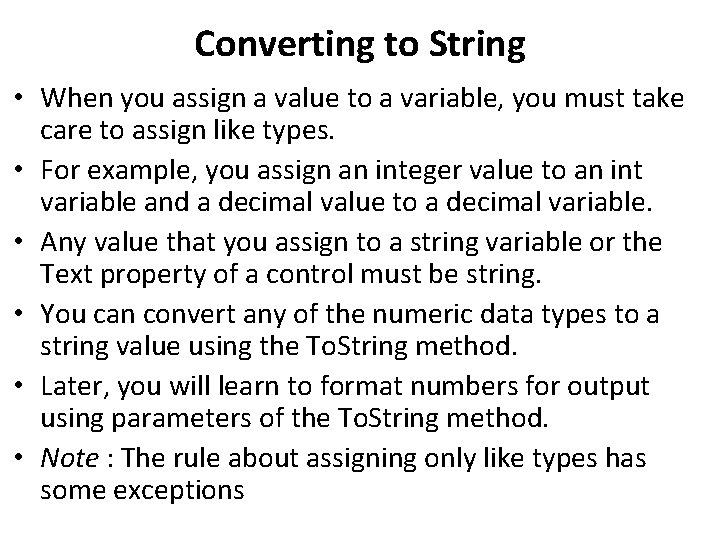Converting to String • When you assign a value to a variable, you must take care to assign like types. • For example, you assign an integer value to an int variable and a decimal value to a decimal variable. • Any value that you assign to a string variable or the Text property of a control must be string. • You can convert any of the numeric data types to a string value using the To. String method. • Later, you will learn to format numbers for output using parameters of the To. String method. • Note : The rule about assigning only like types has some exceptions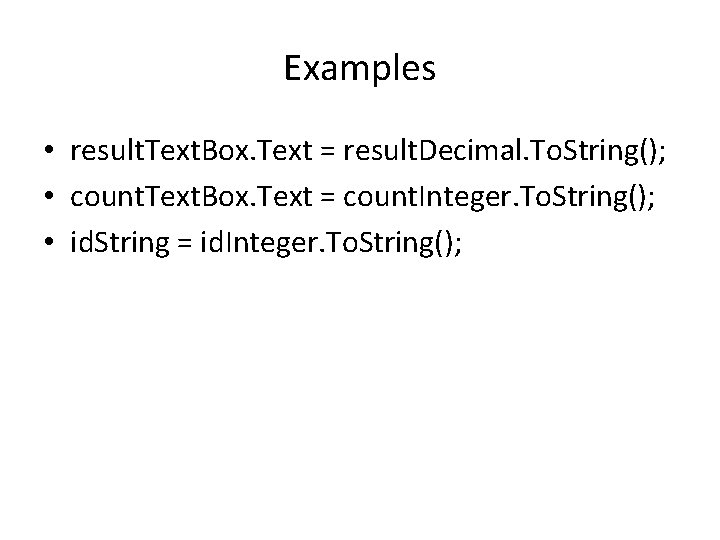Examples • result. Text. Box. Text = result. Decimal. To. String(); • count. Text. Box. Text = count. Integer. To. String(); • id. String = id. Integer. To. String();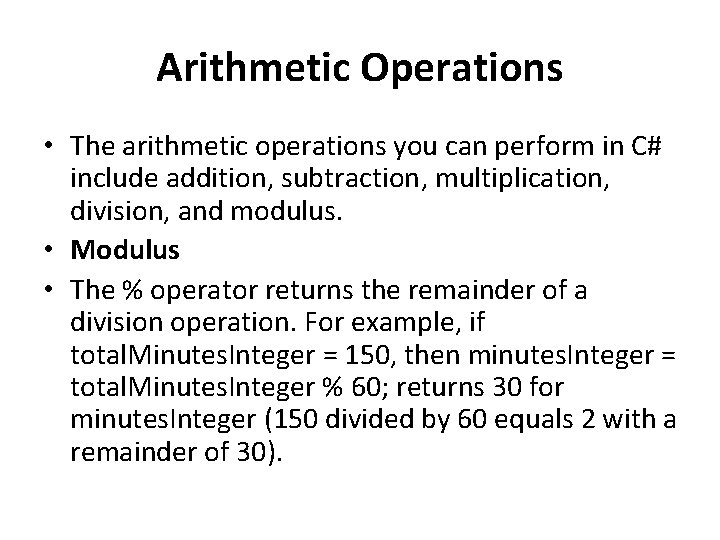Arithmetic Operations • The arithmetic operations you can perform in C# include addition, subtraction, multiplication, division, and modulus. • Modulus • The % operator returns the remainder of a division operation. For example, if total. Minutes. Integer = 150, then minutes. Integer = total. Minutes. Integer % 60; returns 30 for minutes. Integer (150 divided by 60 equals 2 with a remainder of 30).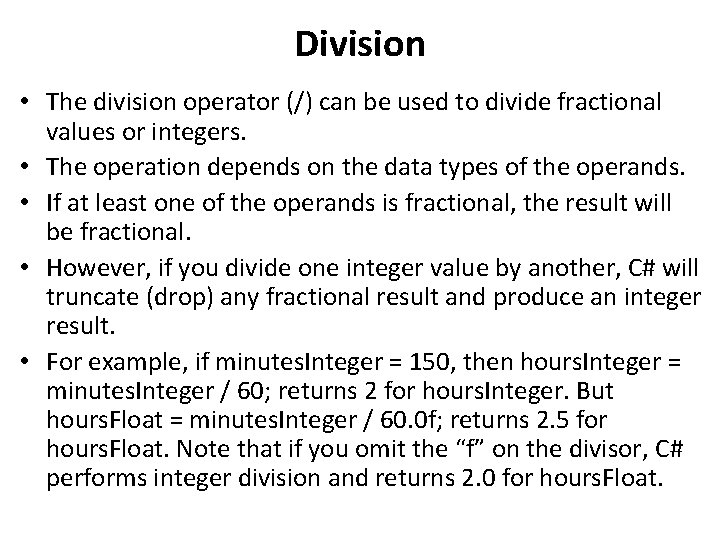Division • The division operator (/) can be used to divide fractional values or integers. • The operation depends on the data types of the operands. • If at least one of the operands is fractional, the result will be fractional. • However, if you divide one integer value by another, C# will truncate (drop) any fractional result and produce an integer result. • For example, if minutes. Integer = 150, then hours. Integer = minutes. Integer / 60; returns 2 for hours. Integer. But hours. Float = minutes. Integer / 60. 0 f; returns 2. 5 for hours. Float. Note that if you omit the “f” on the divisor, C# performs integer division and returns 2. 0 for hours. Float.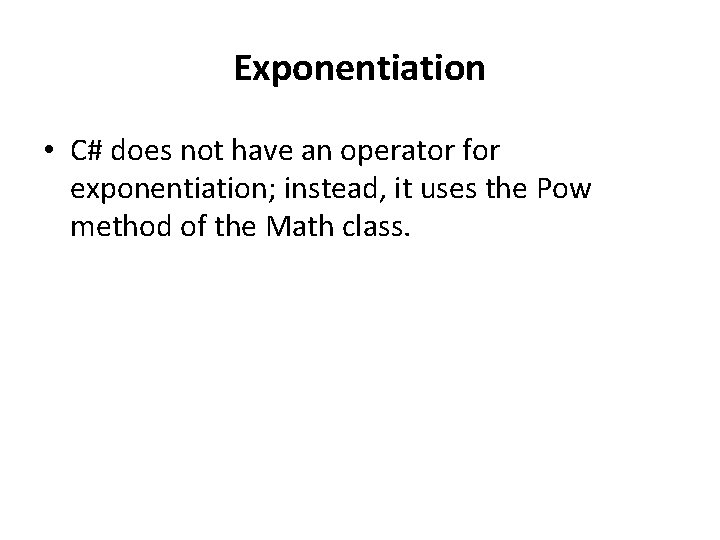Exponentiation • C# does not have an operator for exponentiation; instead, it uses the Pow method of the Math class.Order of Operations • The order in which operations are performed determines the result. Consider the expression 3 + 4 * 2. What is the result? • If the addition is done first, the result is 14. However, if the multiplication is done first, the result is 11. • The hierarchy of operations, or order of precedence , in arithmetic expressions from highest to lowest is 1. Any operation inside parentheses. 2. Multiplication and division. 3. Modulus. 4. Addition and subtraction • In the previous example, the multiplication is performed before the addition, yielding a result of 11. To change the order of evaluation, use parentheses.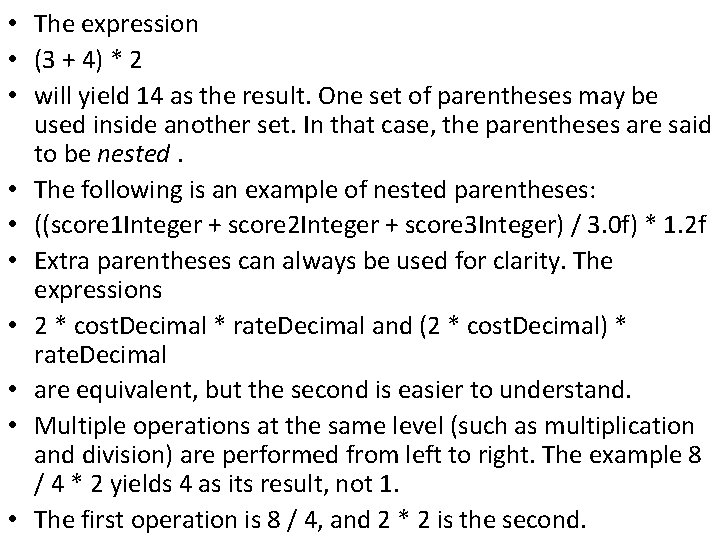• The expression • (3 + 4) * 2 • will yield 14 as the result. One set of parentheses may be used inside another set. In that case, the parentheses are said to be nested. • The following is an example of nested parentheses: • ((score 1 Integer + score 2 Integer + score 3 Integer) / 3. 0 f) * 1. 2 f • Extra parentheses can always be used for clarity. The expressions • 2 * cost. Decimal * rate. Decimal and (2 * cost. Decimal) * rate. Decimal • are equivalent, but the second is easier to understand. • Multiple operations at the same level (such as multiplication and division) are performed from left to right. The example 8 / 4 * 2 yields 4 as its result, not 1. • The first operation is 8 / 4, and 2 * 2 is the second.• Evaluation of an expression occurs in this order: 1. All operations within parentheses. Multiple operations within the parentheses are performed according to the rules of precedence. 2. All multiplication and division. Multiple operations are performed from left to right. 3. Modulus operations. Multiple operations are performed from left to right. 4. All addition and subtraction are performed from left to right. Although the precedence of operations in C# is the same as in algebra, take note of one important difference: There are no implied operations in C#.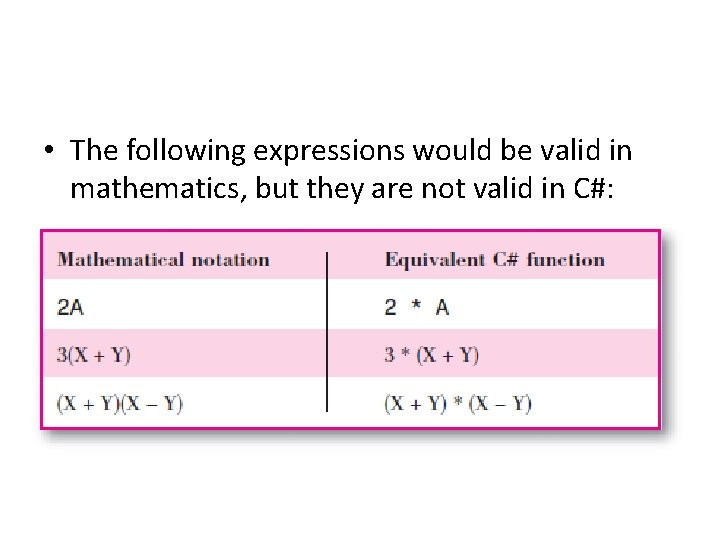• The following expressions would be valid in mathematics, but they are not valid in C#: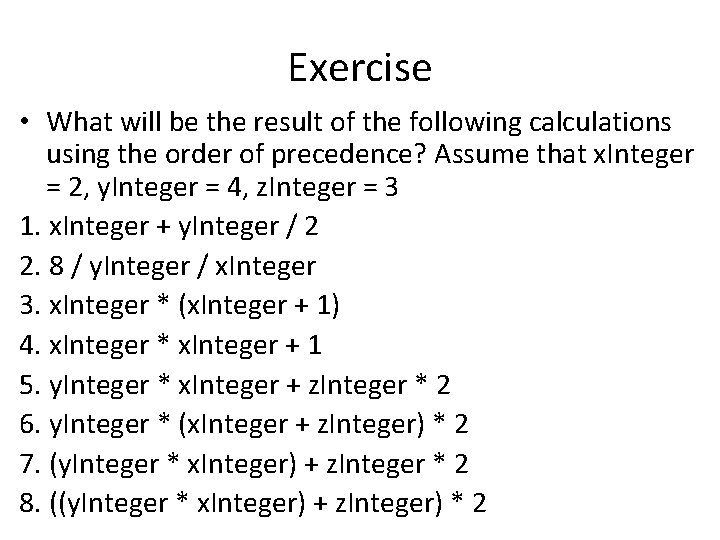Exercise • What will be the result of the following calculations using the order of precedence? Assume that x. Integer = 2, y. Integer = 4, z. Integer = 3 1. x. Integer + y. Integer / 2 2. 8 / y. Integer / x. Integer 3. x. Integer * (x. Integer + 1) 4. x. Integer * x. Integer + 1 5. y. Integer * x. Integer + z. Integer * 2 6. y. Integer * (x. Integer + z. Integer) * 2 7. (y. Integer * x. Integer) + z. Integer * 2 8. ((y. Integer * x. Integer) + z. Integer) * 2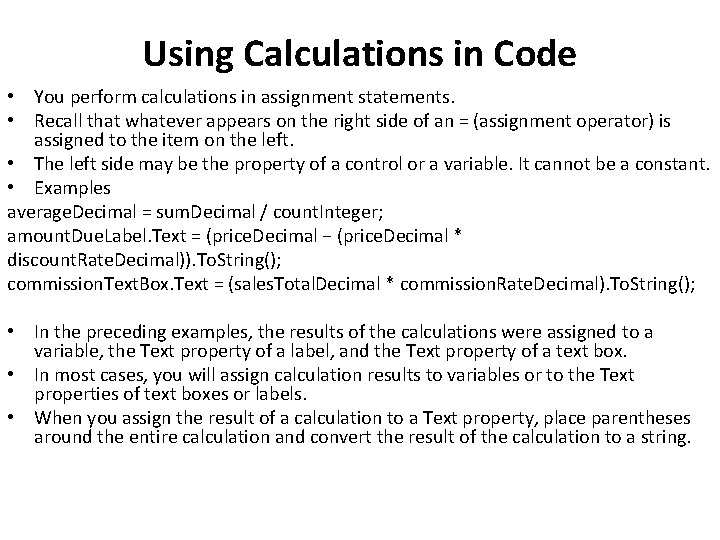Using Calculations in Code • You perform calculations in assignment statements. • Recall that whatever appears on the right side of an = (assignment operator) is assigned to the item on the left. • The left side may be the property of a control or a variable. It cannot be a constant. • Examples average. Decimal = sum. Decimal / count. Integer; amount. Due. Label. Text = (price. Decimal − (price. Decimal * discount. Rate. Decimal)). To. String(); commission. Text. Box. Text = (sales. Total. Decimal * commission. Rate. Decimal). To. String(); • In the preceding examples, the results of the calculations were assigned to a variable, the Text property of a label, and the Text property of a text box. • In most cases, you will assign calculation results to variables or to the Text properties of text boxes or labels. • When you assign the result of a calculation to a Text property, place parentheses around the entire calculation and convert the result of the calculation to a string.Assignment Operators • In addition to the equal sign (=) as an assignment operator , C# has several operators that can perform a calculation and assign the result as one operation. • The combined assignment operators are +=, – =, *=, /=, %=, and += (string). • Each of these combined assignment operators is a shortcut for the standard method; you can use the standard (longer) form or the shortcut. • The shortcuts allow you to type a variable name only once instead of having to type it on both sides of the equal sign.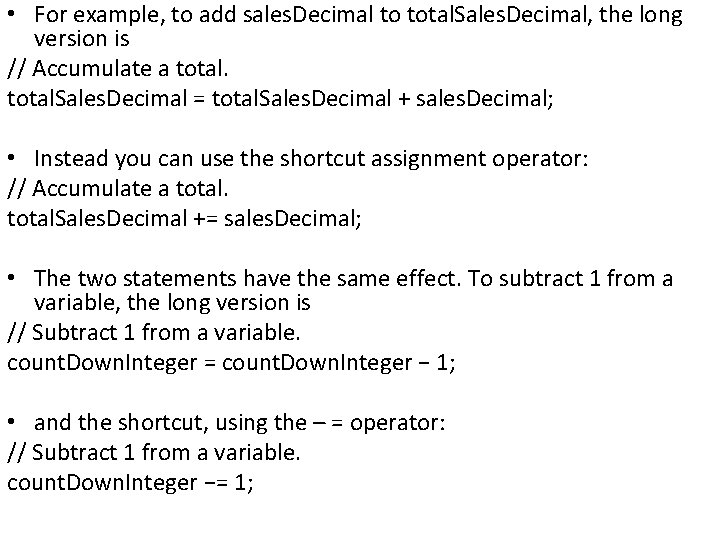• For example, to add sales. Decimal to total. Sales. Decimal, the long version is // Accumulate a total. Sales. Decimal = total. Sales. Decimal + sales. Decimal; • Instead you can use the shortcut assignment operator: // Accumulate a total. Sales. Decimal += sales. Decimal; • The two statements have the same effect. To subtract 1 from a variable, the long version is // Subtract 1 from a variable. count. Down. Integer = count. Down. Integer − 1; • and the shortcut, using the – = operator: // Subtract 1 from a variable. count. Down. Integer −= 1;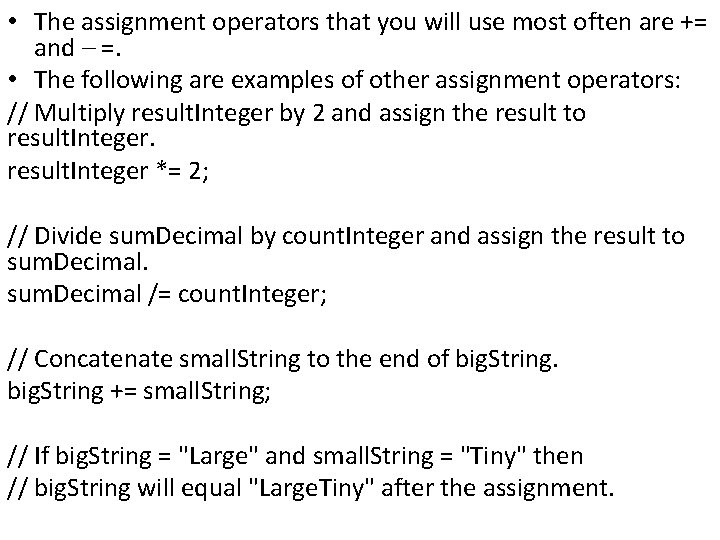• The assignment operators that you will use most often are += and – =. • The following are examples of other assignment operators: // Multiply result. Integer by 2 and assign the result to result. Integer *= 2; // Divide sum. Decimal by count. Integer and assign the result to sum. Decimal /= count. Integer; // Concatenate small. String to the end of big. String += small. String; // If big. String = "Large" and small. String = "Tiny" then // big. String will equal "Large. Tiny" after the assignment.